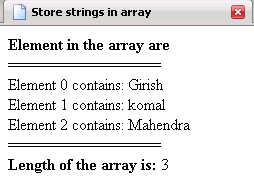Tutorials

# JavaScript array method

In This Tutorial we want to describe you a code that help you in understanding JavaScript Method For this method we have JavaScript as script language.

In This Tutorial we want to describe you a code that help you in understanding JavaScript Method For this method we have JavaScript as script language.

# JavaScript array method

In This Tutorial we want to describe you a code that help you in understanding JavaScript Method For this method we have JavaScript as script language. We instantiate an array instance and assign to variable array. Each  component in an array is accessed by an array expression that consists of an expression whose value is an array reference indicated by subscripts indexing expression enclosed by []. The document. write print the element in an array followed by splitter line. The for loop run the scripts till the array variable is less than array length. Finally the document. write print the length of an array element by calling array. length( ) method.

ArrayLength.html

 ``` Javascript array method var array = new Array(); array = "Girish"; array = "komal"; array = "Mahendra"; document.write(""+"Element in the array are "+""+ ""); document.write("================="+ ""); for (var i=0; i"); } document.write("================="+ ""); document.write(""+"Length of the array is: "+""+array.length); ```

Output of the program